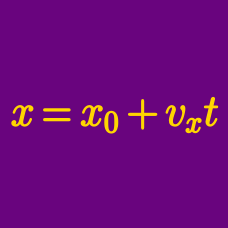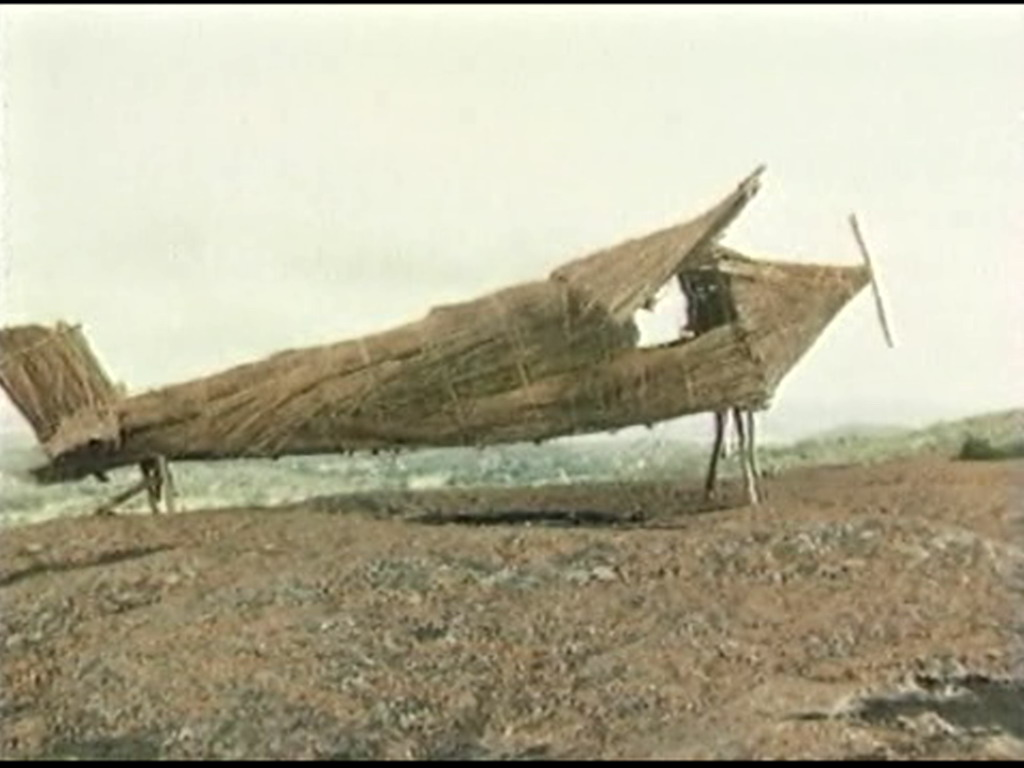Classical Mechanics

# 2D Kinematics - Problem SolvingAn airplane is taking off on the runway. At the moment the wheels leave the ground, the plane is traveling at $$60 \text{ m/s}$$ horizontally. The wings generate a lift which causes a vertical acceleration of $$1 \text{ m/s}^2 ,$$ and the jets produce a horizontal acceleration of $$2 \text{ m/s}^2 .$$ When the airplane is flying at an altitude of $$3200 \text{ m},$$ what is its horizontal distance from the airport?

The air resistance is negligible.

A hunter aims directly at a target $$66 \text{ m}$$ away, at the same height as the gun. If the bullet is fired at a speed of $$66 \text{ m/s},$$ by how much will the bullet miss the target?

NOTES: The air resistance is negligible, and the gravitational acceleration is $$g=10\text{ m/s}^2.$$ You may also assume the bullet does not hit the ground until after passing the target.

A boy throws a ball at $$32 \sqrt{2} \text{ m/s}$$ at a $$45^ \circ$$ angle with the ground. Find the horizontal distance the ball flies.

Gravitational acceleration is $$g =10 \text{ m/s}^2,$$ and air resistance is negligible.

A ball is thrown horizontally at $$16 \text{ m/s} .$$ If the ball travels a horizontal distance of $$80 \text{ m}$$ before hitting the ground, from what height was the ball thrown?

The gravitational acceleration is $$g= 10 \text{ m/s}^2.$$

A car initially at rest accelerates uniformly at a rate of $$(6 \text{ m/s}^2) \hat{i}.$$ If the car travels $$(108 \text{ m}) \hat{i} ,$$ what is its final velocity?

×